white
Performs White’s test for heteroskedasticity of residuals.
Carries out White’s multivariate test for heteroskedasticity of the residuals of the specified Var object. By default, the test is computed without the cross-product terms (using only the terms involving the original variables and squares of the original variables). You may elect to compute the original form of the White test that includes the cross-products.
Syntax
var_name.white(options)
Options

 c Include all possible nonredundant cross-product terms in the test regression. name=arg Save test statistics in named matrix object. See below for a description of the statistics stored in the matrix. p Print the test results.
The “name=” option stores the results in a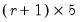matrix, where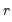is the number of unique residual cross-product terms. For a VAR with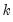endogenous variables,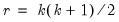. The firstrows contain statistics for each individual test equation, where the first column is the regression R-squared, the second column is the F-statistic, the third column is the p-value of F-statistic, the 4th column is the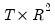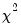statistic, and the fifth column is the p-value of thestatistic.
The numerator and denominator degrees of freedom of the F-statistic are stored in the third and fourth columns, respectively, of the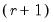-st row, while thedegrees of freedom is stored in the fifth column of the-st row.
In the-st row and first column contains the joint (system) LM chi-square statistic and the second column contains the degrees of freedom of thisstatistic.
Examples
var1.white
carries out the White test of heteroskedasticity.
Cross-references
See “White's Heteroskedasticity Test” for a discussion of White’s test. For the multivariate version of this test, see “White Heteroskedasticity Test”.